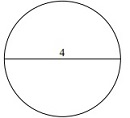# Circumference and Area of a Circle

In this lesson, we are required to find both the circumference and the area of the circle given its radius or diameter.

Formulas to find the circumference and the area of a circle

• Circumference of a circle is given by the formula C = 2πr where π = 3.14 and r is the radius of the circle

• Area of a circle is given by the formula A = πr2 where π = 3.14 and r is the radius of the circle.

Find the area and circumference of following circle. Use π = 3.14### Solution

Step 1:

Given radius r = 9.5 units

Step 2:

Circumference of Circle, C = 2πr = 2(3.14)9.5 = 59.66 units

Step 3:

Area of Circle, A = πr2 = (3.14)(9.5)(9.5) = 283.39 square units

Find the area and circumference of following circle. Use π = 3.14### Solution

Step 1:

Given diameter = 4; radius r = 4/2 = 2 units

Step 2:

Circumference of Circle, C = 2πr = 2(3.14)2 = 12.56 units

Step 3:

Area of Circle, A = πr2 = (3.14)(2)(2) = 12.56 square units